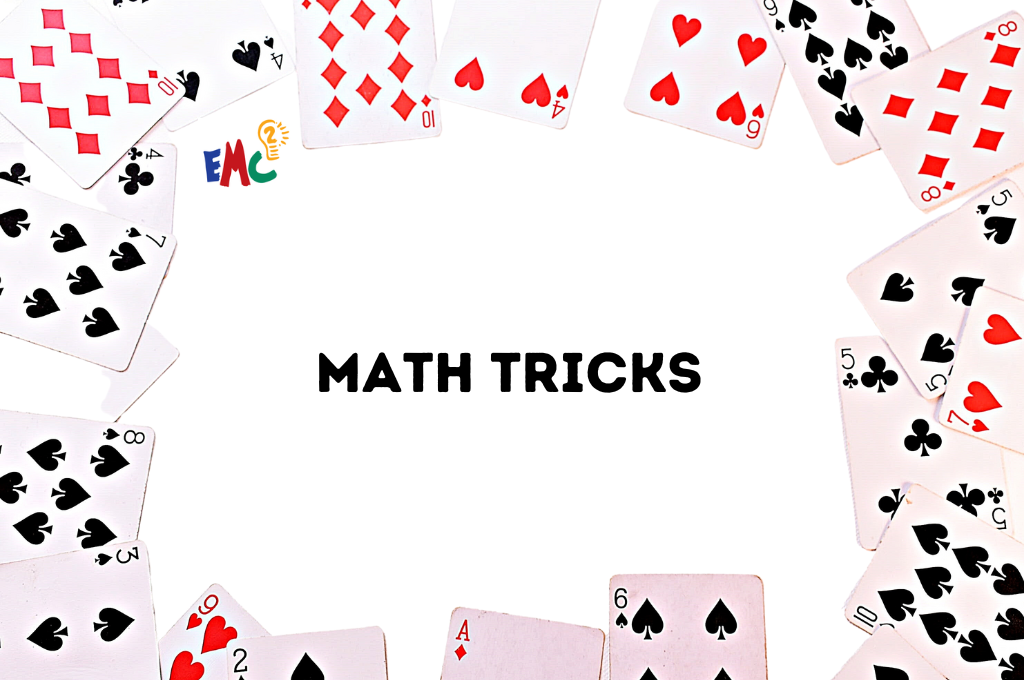# Unlocking the Magic of Math: 10 Mind-Bending Card TricksGet ready to be amazed as we dive into the world of math and cards! We'll reveal 10 incredible math tricks you can do with a standard deck of cards. From building your mental math skills to mastering probability and fractions, these tricks are fun and great for boosting your math and logic muscles. Join us on a journey where numbers and cards come together to create magic right before your eyes!

1. Card Counting:

Shuffle a deck and practice counting cards as they are dealt. This can help improve your mental math skills.

1. Multiplying Pairs:

Pick two cards, multiply the numbers on them, and try to do it quickly. Great for mental multiplication practice.

Lay out cards in rows, then quickly add the values in each row to practice addition.

1. Probability Practice:

Calculate the probability of drawing specific cards or combinations (e.g., drawing two red cards in a row).

1. Estimation Games:

Estimate the total value of a shuffled deck without counting every card. Then check your estimate.

1. Card Tricks:

Learn and perform card tricks that involve math principles, like the "Magic 21" trick.

1. Fractions with Cards:

Create fractions by dividing the deck into parts and calculating the fraction of the total.

1. Prime Number Hunt:

Identify prime-numbered cards (2, 3, 5, 7) as quickly as possible from a shuffled deck.

1. Simple Equations:

Deal two cards and challenge yourself to make equations (addition, subtraction, multiplication, division) using those numbers.

1. Card Combinations:

Calculate the number of possible combinations or permutations of cards in a deck.

“ It's time to unlock the secrets of math, one card at a time!”

But here's the best part: these card tricks are not confined to your living room. They're perfect for those on-the-go moments, whether you're on a road trip, traveling, or simply looking for a bit of mental stimulation. It's a unique blend of entertainment and education that you can take with you anywhere.

Want to discover how numbers and cards combine to create pure magic?

Download this tutorial for more math tricks and games with cards and try solving with your kids.

### Let us know where to send the file:

PDF sent to your mail.  We hope you enjoy the games and tricks!
Oops! Something went wrong while submitting the form.